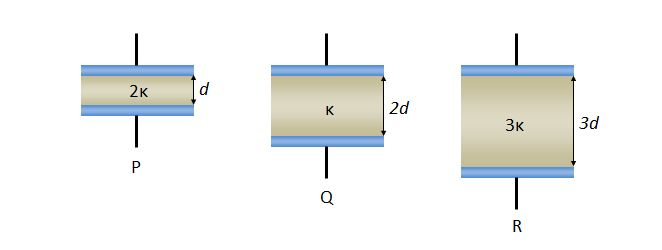# Dielectrics in capacitorsIn the above diagram, there are three capacitors P, Q and R with their respective distinct dielectric materials. The dielectric constant and the thickness of the dielectric material for P, Q and R are $(2\kappa, d), (\kappa, 2d)$ and $(3\kappa, 3d),$ respectively. Let $C_P,$ $C_Q$ and $C_R$ denote the electric capacities of the three capacitors, respectively, then what is the ratio $C_P:C_Q:C_R?$

The plate areas of the three capacitors are the same.

×